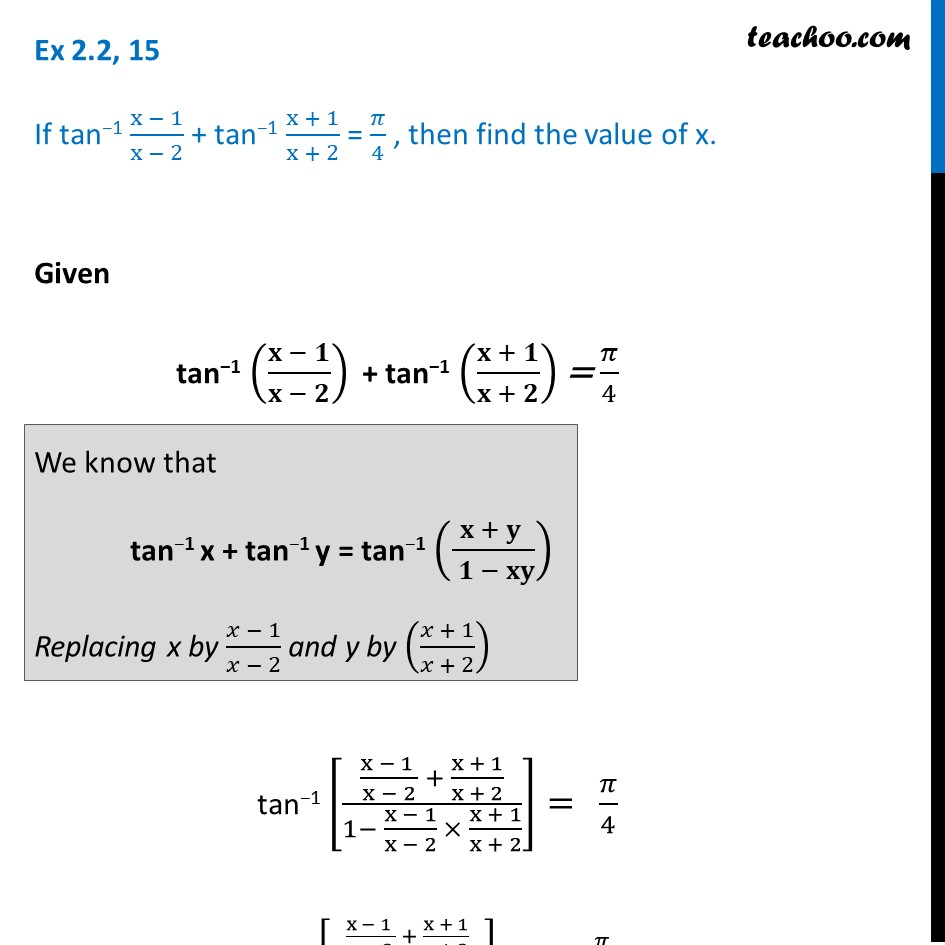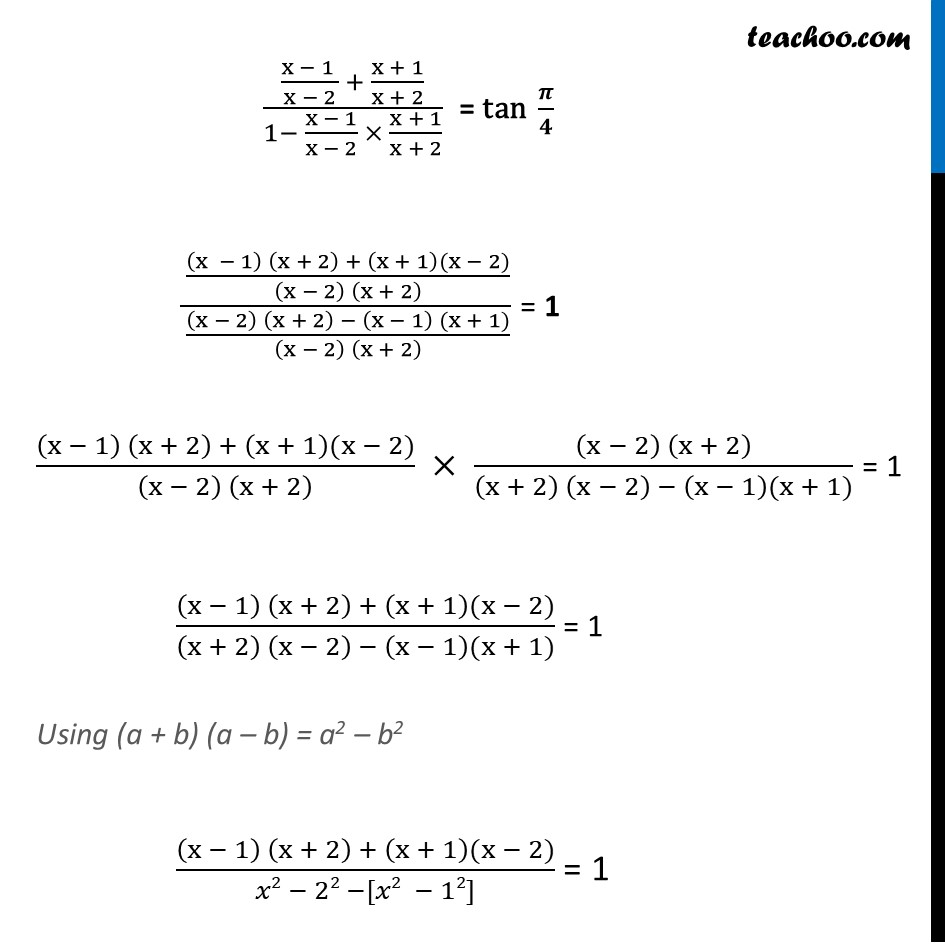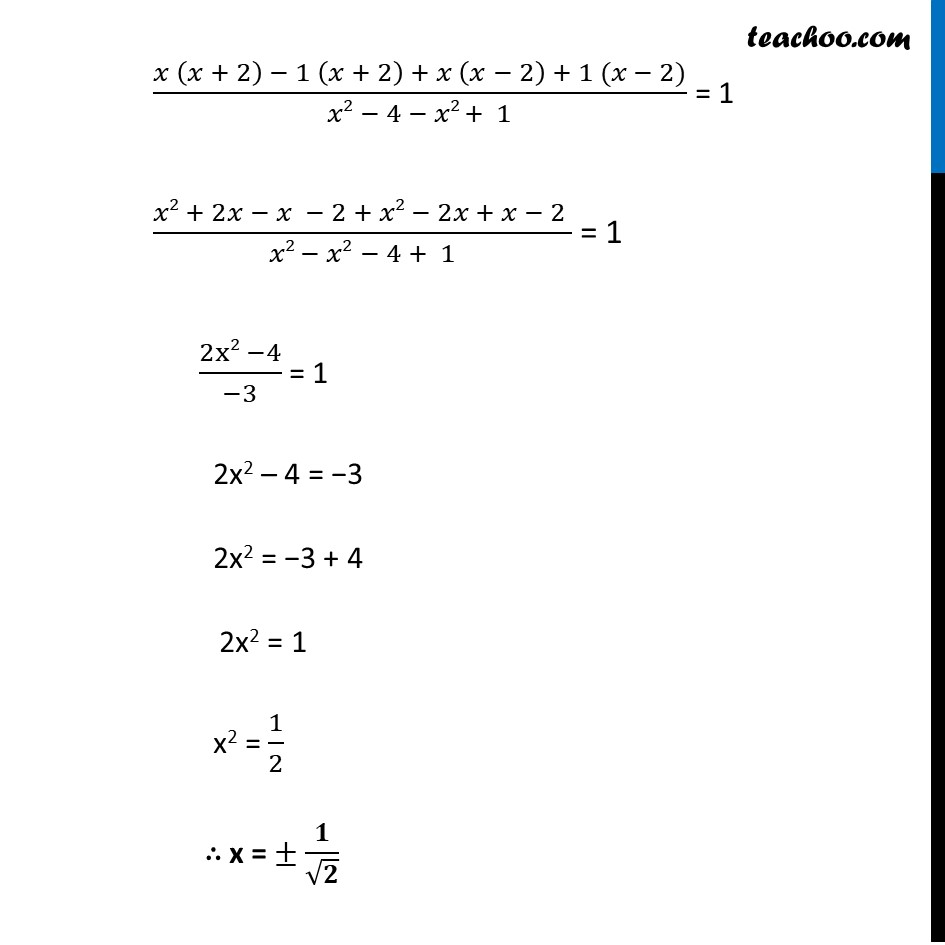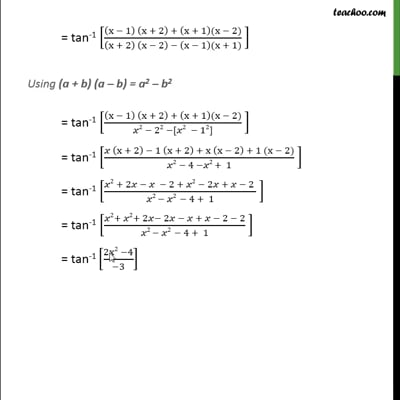Formulae based

Chapter 2 Class 12 Inverse Trigonometric Functions
Concept wiseThis video is only available for Teachoo black users

Solve all your doubts with Teachoo Black (new monthly pack available now!)

### Transcript

Ex 2.2, 15 If tan−1 (x − 1)/(x − 2) + tan−1 (x + 1)/(x + 2) = 𝜋/4 , then find the value of x. Given tan−1 ((𝐱 − 𝟏)/(𝐱 − 𝟐)) + tan−1 ((𝐱 + 𝟏)/(𝐱 + 𝟐)) = 𝜋/4We know that tan−1 x + tan−1 y = tan−1 ((𝐱 + 𝐲 )/( 𝟏 − 𝐱𝐲)) Replacing x by (𝑥 − 1)/(𝑥 − 2) and y by ((𝑥 + 1)/(𝑥 + 2)) tan−1 [((x − 1 )/(x − 2) + (x + 1)/(x + 2))/(1− (x − 1)/(x − 2) × (x + 1)/(x + 2))]=" " 𝜋/4 tan−1 [((x − 1 )/(x − 2) + (x + 1)/(x + 2))/(1− (x − 1)/(x − 2) × (x + 1)/(x + 2))]="tan " 𝜋/4 = tan-1 [(((x − 1) (x + 2) + (x + 1)(x − 2))/((x − 2) (x + 2) ))/(((x − 2) (x + 2) − (x − 1) (x + 1))/((x − 2) (x + 2) ))]((x − 1 )/(x − 2) + (x + 1)/(x + 2))/(1− (x − 1)/(x − 2) × (x + 1)/(x + 2)) = "tan " 𝝅/𝟒 (((x − 1) (x + 2) + (x + 1)(x − 2))/((x − 2) (x + 2) ))/(((x − 2) (x + 2) − (x − 1) (x + 1))/((x − 2) (x + 2) )) = 1 ((x − 1) (x + 2) + (x + 1)(x − 2))/((x − 2) (x + 2) ) × ((x − 2) (x + 2))/((x + 2) (x − 2) − (x − 1)(x + 1)) = 1 ((x − 1) (x + 2) + (x + 1)(x − 2))/((x + 2) (x − 2) − (x − 1)(x + 1)) = 1 Using (a + b) (a – b) = a2 – b2 ((x − 1) (x + 2) + (x + 1)(x − 2))/(𝑥2 − 22 −[𝑥2 − 12]) = 1 (𝑥 (𝑥 + 2) − 1 (𝑥 + 2) + 𝑥 (𝑥 − 2) + 1 (𝑥 − 2))/(𝑥2 − 4 − 𝑥2 + 1) = 1 (𝑥2 + 2𝑥 − 𝑥 − 2 + 𝑥2 − 2𝑥 + 𝑥 − 2 )/(𝑥2 − 𝑥2 − 4 + 1) = 1 (2x2 −4)/(−3) = 1 2x2 – 4 = −3 2x2 = −3 + 4 2x2 = 1 x2 = 1/2 ∴ x = ± 𝟏/√𝟐